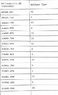# Orbital period of eclipsing binaries

• Sastronaut
In summary, based on the data in the table I want to calculate the orbital period of the eclipsing binary but I want to state my answer in equation form so that any observer can predict the times of future eclipses. Does anyone have an idea on how I go about doing that with the given data. I tried to find a standard equation that I can essentially plug my HJDs into express the period of eclipsing binary. Any ideas would be great. thanks.

#### Sastronaut

based on the data in the table I want to calculate the orbital period of the eclipsing binary but I want to state my answer in equation form so that any observer can predict the times of future eclipses. Does anyone have an idea on how I go about doing that with the given data. I tried to find a standard equation that I can essentially plug my HJDs into express the period of eclipsing binary. Any ideas would be great. thanks pf.

#### Attachments

•23.2 KB · Views: 407
That doesn't look periodic, unless most (like >90%) eclipses are missing for some reason.

As type of the equation, you are probably looking for something like ##t=T_0 + kT## with an offset T0, a period T and an integer k (numbering the eclipses).

the data I was given is ment to be practice for myself so that when I am presented with the full data I will be able to process it. could you show me how to plug my HJD into the equation? what does offset mean as far as my data goes? and if T stands for period what does "t" stand for?

mfb said:
That doesn't look periodic, unless most (like >90%) eclipses are missing for some reason.

As type of the equation, you are probably looking for something like ##t=T_0 + kT## with an offset T0, a period T and an integer k (numbering the eclipses).

okay I read a some stuff online and I understand what you saying. the data I was given is spotty and that was intentional. how can I determine T if I do not know my K value given my offset value (To) equals my secondary minimum 48869.627?

Calculate the time differences, look for something similar to a largest common divisor (not exact, but approximately).
Alternatively, try a Fourier transformation, it might give some interesting results.

If a period T fits, periods T/3, T/5 and so on fit as well - there is no way to resolve that ambiguity, but T is more likely (otherwise you have a very special set of observations).

I never thought to use Fourier transformation for it...though to be honest that maybe over thinking the problem...I feel that the answer is in front of me I just can't figure it out. I calculated differences in HJDs to see what was the lowest value I could get from the difference moving down the list secondary minimum to secondary minimum. I determined that the lowest orbital period I could get with doing this is 40.913HJD which was calculated by subtracting (49899.744-49858.831)HJD. But I agree with you 100%. there is no way to resolve that ambiguity so I am not sure how I will know that the period I calculated is the correct one, and not some multiple of the true period.